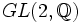# General linear group over subring of field need not be conjugacy-closed

## Statement

It is possible to have a field$K$, a unital subring$R$ of$K$ (note, in particular, that$R$ is an integral domain), and a natural number$n$ such that there exist matrices$A,B \in GL_n(R)$ such that$A$ and$B$ are conjugate in the General linear group (?)$GL_n(K)$ but not in the general linear group$GL_n(R)$.

## Proof

### Example of the integers and the rationals

Let$R$ be the ring of integers$\mathbb{Z}$ and$K$ be the field of rational numbers$\mathbb{Q}$. Consider the matrices:$A = \begin{pmatrix}1 & 1 \\ 0 & 1\end{pmatrix}, B = \begin{pmatrix} 1 & 2 \\ 0 & 1 \end{pmatrix}$

These matrices are conjugate in$GL(2,\mathbb{Q})$, by the matrix:$\begin{pmatrix} 2 & 0 \\ 0 & 1 \end{pmatrix}$.

On the other hand, the matrices are not conjugate in$GL(2,\mathbb{Z})$. (Not ethat although the above matrix has integer entries, it is not in$GL(2,\mathbb{Z})$ because its inverse does not have integer entries).